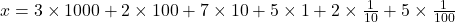## Which number is one tenth of the number shown in expanded form below? (3×1000)+(2×100)+(7×10)+(5×1)+(2x 1/10)+5×1/100

Question

Which number is one tenth of the number shown in expanded form below? (3×1000)+(2×100)+(7×10)+(5×1)+(2x 1/10)+5×1/100

in progress 0
3 weeks 2021-08-25T02:27:15+00:00 1 Answers 0 views 0

Let be, the digit that is one tenth of the number shown in expanded form is that whose numerator that multiplies it is 1 and denominator is 10. Therefore, the digit that is one tenth of the number is 2.